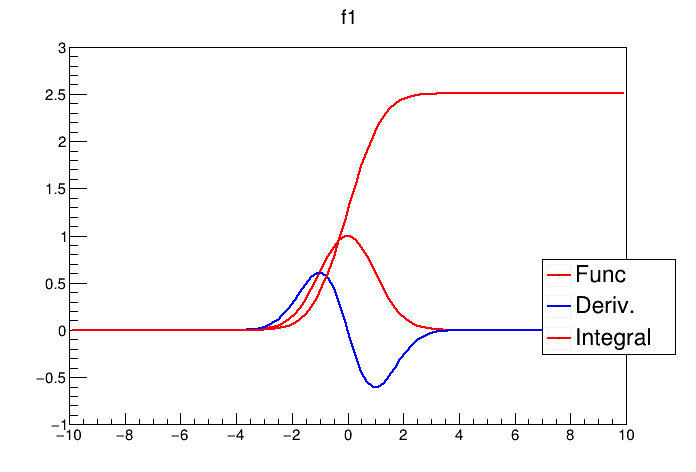# Example Functor¶

Tutorial illustrating how creating a TF1 class using functor or class member functions

can be run with:

root > .x exampleFunctor.C
root > .x exampleFunctor.C+ with ACLIC


Author: Lorenzo Moneta
This notebook tutorial was automatically generated with ROOTBOOK-izer from the macro found in the ROOT repository on Saturday, June 25, 2022 at 08:39 AM.

In :
%%cpp -d
#include "TF1.h"
#include "TMath.h"
#include "TLegend.h"


Function object (functor)

In :
struct MyDerivFunc {
MyDerivFunc(TF1 * f): fFunc(f) {}
double operator() (double *x, double * )  const {
return fFunc->Derivative(*x);
}
TF1 * fFunc;
};


Function class with a member function

In :
struct MyIntegFunc {
MyIntegFunc(TF1 * f): fFunc(f) {}
double Integral (double *x, double * ) const {
double a = fFunc->GetXmin();
return fFunc->Integral(a, *x);
}
TF1 * fFunc;
};


A helper function is created:

In :
%%cpp -d
double MyFunc (double *x, double *p ) {
return TMath::Gaus(x,p,p );
}

In :
double xmin = -10;  double xmax = 10;


Create tf1 using a free c function

In :
TF1 * f1 = new TF1("f1",MyFunc,xmin,xmax,2);
f1->SetParameters(0.,1.);
f1->SetMaximum(3);   f1->SetMinimum(-1);
f1->Draw();

Info in <TCanvas::MakeDefCanvas>:  created default TCanvas with name c1


Derivative function example to create TF1 using a functor

In order to work with interpreter the function object must be created and lived all time for all time of the TF1. In compiled mode, the function object can be passed by value (recommended) and there is also no need to specify the type of the function class. Example is as follows:

TF1 * f2 = new TF1("f2",MyDerivFunc(f1), xmin, xmax,0); // only for C++ compiled mode

In :
MyDerivFunc * deriv = new MyDerivFunc(f1);
TF1 * f2 = new TF1("f2",deriv, xmin, xmax, 0);

f2->SetLineColor(kBlue);
f2->Draw("same");


Integral function example to create a TF1 using a member function of a user class

In order to work with interpreter the function object must be created and lived all time for all time of the TF1. In compiled mode there is no need to specify the type of the function class and the name of the member function

TF1 * f3 = new TF1("f3",intg,&MyIntegFunc::Integral,xmin,xmax, 0); // only for C++ compiled mode

In :
MyIntegFunc * intg = new MyIntegFunc(f1);
TF1 * f3 = new TF1("f3",intg,&MyIntegFunc::Integral, xmin, xmax, 0);

f3->SetLineColor(kRed);
f3->Draw("same");

TLegend * l = new TLegend(0.78, 0.25, 0.97 ,0.45);

gROOT->GetListOfCanvases()->Draw()